## 基于人工弹簧模型的周期结构带隙计算方法研究1)

*华东交通大学 铁路环境振动与噪声教育部工程研究中心, 南昌330013

## RESEARCH ON BAND GAP CALCULATION METHOD OF PERIODIC STRUCTURE BASED ON ARTIFICIAL SPRING MODEL1)

Feng Qingsong*, Yang Zhou*, Guo Wenjie,*,2), Lu Jianfei, Liang Yuxiong*

*Engineering Research Center of Railway Environment Vibration and Noise, Ministry of Education, East China Jiaotong University, Nanchang 330013, China

Faculty of Civil Engineering and Mechanics, Jiangsu University, Zhenjiang 212013, Jiangsu, China

 基金资助: 1)国家自然科学基金.  51878277国家自然科学基金.  52068029江西省主要学科学术和技术带头人培养计划.  20194BCJ22008江西省重点研发计划.  20192BBE50008江西省青年科学基金.  20202BABL214049

Received: 2020-01-6   Accepted: 2021-03-25   Online: 2021-06-18Abstract

Keywords： artificial spring ; computational efficiency ; periodic structure ; band gaps ; energy method

Feng Qingsong, Yang Zhou, Guo Wenjie, Lu Jianfei, Liang Yuxiong. RESEARCH ON BAND GAP CALCULATION METHOD OF PERIODIC STRUCTURE BASED ON ARTIFICIAL SPRING MODEL1). Chinese Journal of Theoretical and Applied Mechanics, 2021, 53(6): 1684-1697 DOI:10.6052/0459-1879-21-007

## 1 人工弹簧模拟周期边界的基本原理

### 图1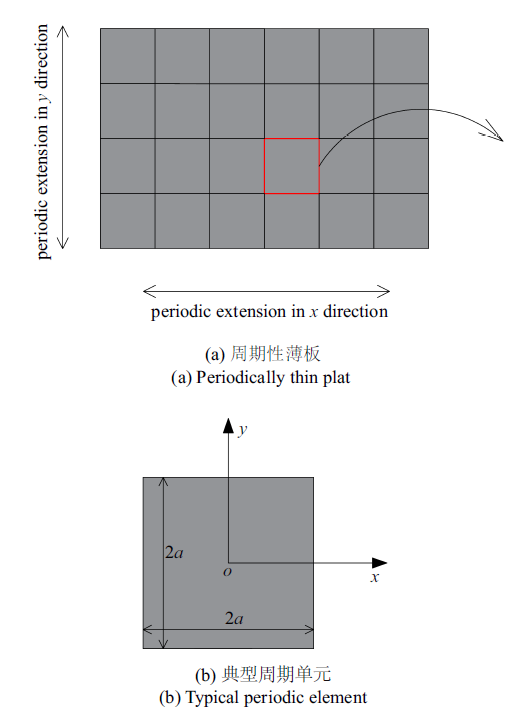Fig.1   Vibration analysis model of a periodically thin plate with free boundary

$[ u \ \ v \ \ w]^T=\left[-z\frac{\partial w}{\partial x} \ \ -z\frac{\partial w}{\partial y} \ \ w\left( {x,y,t} \right) \right]^T$

$\left. \begin{array}{l} w\left( {x,y,t} \right)= \sum_i {d_{i} \left( t \right)\xi_{i} \left( {x,y} \right)} =\\\qquad d^T\xi =\xi^T d, \ \ \xi =\varphi \otimes \psi\\ \left.\begin{array}{l} \varphi =\left[ {\varphi_{1} \left( x \right),\varphi_{2} \left( x \right),\cdots ,\varphi_{i} \left( x \right),\cdots ,\varphi_{m} \left( x \right)} \right]^T \\ \psi =\left[ {\psi_{1} \left( y \right),\psi_{2} \left( y \right),\cdots ,\psi_{i} \left( y \right),\cdots ,\psi_{n} \left( y \right)} \right]^T \\ \end{array} \right. \\ \end{array} \right\}$

$w ( {-a,y} )=w( {a,y} )e^{-ik_{x} 2a}, {w( {x,-a} )=w( {x,a} )e^{-ik_{y} 2a}}$
$\left.\begin{array}{l} {\dfrac{\partial w\left( {-a,y} \right)}{\partial x}=\dfrac{\partial w\left({a,y} \right)}{\partial x}e^{-ik_{x} 2a}} \\ {\dfrac{\partial w\left({x,-a} \right)}{\partial y}=\dfrac{\partial w\left( {x,a} \right)}{\partial y}e^{-ik_{y} 2a}} \\ \end{array} \right\}$
$\left.\begin{array}{l} {\dfrac{\partial ^{2}w\left( {-a,y} \right)}{\partial x^{2}}=\dfrac{\partial ^{2}w\left( {a,y} \right)}{\partial x^{2}}e^{-ik_{x} 2a}}\\ {\dfrac{\partial ^{2}w\left( {x,-a} \right)}{\partial y^{2}}=\dfrac{\partial ^{2}w\left( {x,a} \right)}{\partial y^{2}}e^{-ik_{y} 2a}} \\ \end{array} \right\}$
$\left.\begin{array}{l} {\dfrac{\partial ^{3}w\left( {-a,y} \right)}{\partial x^{3}}=-\dfrac{\partial ^{3}w\left( {a,y} \right)}{\partial x^{3}}e^{-ik_{x} 2a}} \\ {\dfrac{\partial ^{3}w\left( {x,-a} \right)}{\partial y^{3}}=-\dfrac{\partial ^{3}w\left( {x,a} \right)}{\partial y^{3}}e^{-ik_{y} 2a}} \\ \end{array} \right\}$

\begin{align} E_{k}^{\ast } =\frac{1}{2}\int_{-a}^a {\int_{-a}^a {\rho h\dot{{w}}^{2}dxdy} } = \\ \frac{1}{2}\dot{{ d}}^{H}\Bigg[ \int_{-a}^a \int_{-a}^a \rho h \xi^{{\ast }}\left( {k_{x} ,k_{y} ,x,y} \right)\xi^{\ast H}\cdot \\ \left( {k_{x} ,k_{y} ,x,y} \right)dxdy \Bigg]\dot{{ d}}= \frac{1}{2}\dot{{ d}}^{H} M^{{\ast }}\left( {k_{x} ,k_{y} } \right)\dot{{ d}} \end{align}
\begin{align} U_{p}^{\ast } =\frac{1}{2}\int_{-a}^a \int_{-a}^a D\Bigg[ \left( {\frac{\partial ^{2}w}{\partial x^{2}}} \right)^{2}+\left( {\frac{\partial ^{2}w}{\partial y^{2}}} \right)^{2}+ \\ \nu \Bigg( \frac{\partial ^{2}w}{\partial x^{2}}\frac{\partial ^{2}w}{\partial y^{2}} +\frac{\partial ^{2}w}{\partial y^{2}}\frac{\partial ^{2}w}{\partial x^{2}} \Bigg)+ \\ 2\left( {1-\nu } \right)\left( {\frac{\partial ^{2}w}{\partial x\partial y}} \right)^{2} \Bigg]dxdy = \\ \frac{1}{2} d^{H}\{\int_{-a}^a \int_{-a}^a D[\frac{\partial ^{2}\xi^{{\ast }}\left( {k_{x} ,k_{y} ,x,y} \right)}{\partial x^{2}}\cdot \\ \frac{\partial ^{2} \xi^{\ast H}\left( {k_{x} ,k_{y} ,x,y} \right)}{\partial x^{2}}+\frac{\partial ^{2} \xi^{{\ast }}\left( {k_{x} ,k_{y} ,x,y} \right)}{\partial y^{2}}\cdot \\ \frac{\partial ^{2} \xi^{\ast H}\left( {k_{x} ,k_{y} ,x,y} \right)}{\partial y^{2}} +\nu ( \frac{\partial ^{2} \xi^{{\ast }}\left( {k_{x} ,k_{y} ,x,y} \right)}{\partial x^{2}}\cdot \\ \frac{\partial ^{2} \xi^{\ast H}\left( {k_{x} ,k_{y} ,x,y} \right)}{\partial y^{2}}+\frac{\partial ^{2} \xi^{{\ast }}\left( {k_{x} ,k_{y} ,x,y} \right)\xi }{\partial y^{2}}\cdot \\ \frac{\partial ^{2} \xi^{\ast H}\left( {k_{x} ,k_{y} ,x,y} \right)}{\partial x^{2}} ) +2\left( {1-\nu } \right)\frac{\partial ^{2} \xi^{{\ast }}\left( {k_{x} ,k_{y} ,x,y} \right)}{\partial x\partial y}\cdot \\ \frac{\partial ^{2} \xi ^{\ast H}\left( {k_{x} ,k_{y} ,x,y} \right)}{\partial y\partial x} ]dxdy \} d =\frac{1}{2} d^{H} K^{{\ast }}\left( k_{x} ,k_{y} \right) d \end{align}

$L=E_{k}^{\ast } -U_{p}^{\ast } =\frac{1}{2}\dot{{ d}}^{H} M^{{\ast }}\left( {k_{x} ,k_{y} } \right)\dot{{ d}}-\frac{1}{2} d^{H} K^{{\ast }}\left( {k_{x} ,k_{y} } \right) d$

$\frac{\partial }{\partial t}\left( {\frac{\partial L}{\partial \dot{{d}}}} \right)-\frac{\partial L}{\partial d}=0$

$\left[ {K^{{\ast }}\left( {k_{x} ,k_{y} } \right)-\omega ^{2}M^{{\ast }}\left( {k_{x} ,k_{y} } \right)} \right] \overset{\frown }{\mathop{D}} ={\bf 0}$

### 图2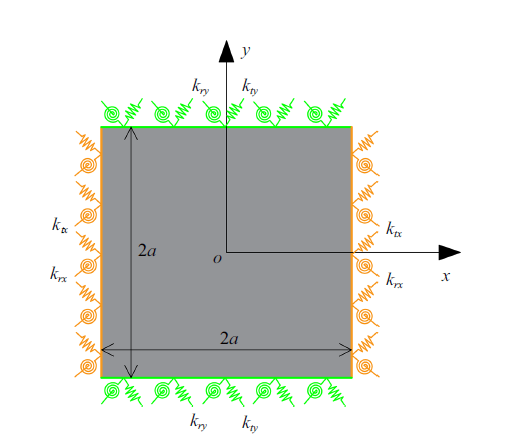Fig.2   Analysis schematic diagram of periodic boundary defined by artificial spring

$U_{p}^{(p_{x} )} =\frac{1}{2}\int_{-a}^a \Bigg\{k_{tx} \left[ {w\left( {-a,y} \right)-w\left( {a,y} \right)e^{-ik_{x} 2a}} \right]^{2} + \\ k_{rx} \left[ \frac{\partial w\left( {-a,y} \right)}{\partial x}-\frac{\partial w\left( {a,y} \right)}{\partial x}e^{-ik2a} \right]^{2} \Bigg\}dy$

$\left. \begin{array}{l} \lim \left[ {w\left( {-a,y} \right)-w\left( {a,y} \right)e^{-ik_{x} 2a}}\right]=\\\qquad \lim \left( {-\dfrac{F}{k_{tx} }} \right)=0 \\ \lim \left[ {\dfrac{\partial w\left( {-a,y} \right)}{\partial x}-\dfrac{\partial w\left( {a,y} \right)}{\partial x}e^{-ik2a}} \right]=\\\qquad \lim\left( {-\dfrac{M}{k_{tx} }} \right)=0 \\ \end{array} \right\}$

\begin{align} U_{p}^{(p_{xy} )} =\frac{1}{2}\Bigg\{\int_{-a}^a \Bigg\{ k_{tx} \left[ {w\left( {-a,y} \right)-w\left( {a,y} \right)e^{-ik_{x} 2a}} \right]^{2} + \\ k_{rx} \left[ {\frac{\partial w\left( {-a,y} \right)}{\partial x}-\frac{\partial w\left( {a,y} \right)}{\partial x}e^{-ik2a}} \right]^{2}dy \Bigg\} + \\ \int_{-a}^a \Bigg\{ k_{ty} \left[ {w\left( {x,-a} \right)-w\left( {x,a} \right)e^{-ik_{y} 2a}} \right]^{2} + \\ k_{ry} \left[ {\frac{\partial w\left( {x,-a} \right)}{\partial y}-\frac{\partial w\left( {x,a} \right)}{\partial y}e^{-ik_{y} 2a}} \right]^{2}dx \Bigg\}\Bigg\} = \\ \frac{1}{2} d^{H}\Bigg\{\int_{-a}^a \Bigg\{k_{tx} \left[ {\xi \left( {-a,y} \right)-\xi \left( {a,y} \right)e^{-ik_{x} 2a}} \right]^{2}+ \\ k_{rx} \left[ {\frac{\partial \xi \left( {-a,y} \right)}{\partial x}-\frac{\partial \xi \left( {a,y} \right)}{\partial x}e^{-ik_{x} 2a}} \right]^{2}dy\Bigg\} + \\ \int_{-a}^a \Bigg\{ k_{ty} \left[ {\xi \left( {x,-a} \right)-\xi \left( {x,a} \right)e^{-ik_{y} 2a}} \right]^{2} + \\ k_{ry} \left[ {\frac{\partial \xi \left( {x,-a} \right)}{\partial y}-\frac{\partial \xi \left( {x,a} \right)}{\partial y}e^{-ik_{y} 2a}} \right]^{2}dx\Bigg\}\Bigg\} d = \\ \frac{1}{2} d^{H}K_periodic \left( {k_{x} ,k_{y} } \right) d \end{align}

$E_{k} =\frac{1}{2}\int_{-a}^a {\int_{-a}^a {\rho h\dot{{w}}^{2}dxdy} } = \\ \frac{1}{2}\dot{{ d}}^{H}\left[ {\int_{-a}^a {\int_{-a}^a {\rho h\xi \left( {x,y} \right)\xi ^{H}\left( {x,y} \right)dxdy} } } \right]\dot{{ d}}= \frac{1}{2}\dot{{ d}}^{H} M\dot{{ d}}$
\begin{align} U_{p} =\frac{1}{2}\int_{-a}^a \int_{-a}^a D\Bigg[ \left( {\frac{\partial ^{2}w}{\partial x^{2}}} \right)^{2}+\left( {\frac{\partial ^{2}w}{\partial y^{2}}} \right)^{2}+ \\ \nu \left( \frac{\partial ^{2}w}{\partial x^{2}}\frac{\partial ^{2}w}{\partial y^{2}} +\frac{\partial ^{2}w}{\partial y^{2}}\frac{\partial ^{2}w}{\partial x^{2}} \right)+ \\ 2\left( {1-\nu } \right)\left( {\frac{\partial ^{2}w}{\partial x\partial y}} \right)^{2} \Bigg]dxdy = \\ \frac{1}{2} d^{H}\Bigg\{ \int_{-a}^a \int_{-a}^a D\Bigg[ \frac{\partial ^{2}\xi \left( {x,y} \right)}{\partial x^{2}}\frac{\partial ^{2}\xi^{^{H}}\left( {x,y} \right)}{\partial x^{2}}+ \\ \frac{\partial ^{2}\xi \left( {x,y} \right)}{\partial y^{2}}\frac{\partial ^{2}\xi ^{^{H}}\left( {x,y} \right)}{\partial y^{2}} +\nu \Bigg( \frac{\partial ^{2}\xi \left( {x,y} \right)}{\partial x^{2}}\frac{\partial ^{2}\xi^{^{H}}\left( {x,y} \right)}{\partial y^{2}}+ \\ \frac{\partial ^{2}\xi \left( {x,y} \right)\xi }{\partial y^{2}}\frac{\partial ^{2}\xi^{^{H}}\left( {x,y} \right)}{\partial x^{2}} \Bigg) +2\left( {1-\nu } \right)\frac{\partial ^{2}\xi \left( {x,y} \right)}{\partial x\partial y}\cdot \\ \frac{\partial ^{2}\xi^{^{H}}\left( {x,y} \right)}{\partial y\partial x}\Bigg]dxdy \Bigg\} d =\frac{1}{2} d^{H} K d \end{align}

$L=E_{k} -U_{p} -U_{p}^{(p_{xy} )} =\frac{1}{2}\dot{{ d}}^{H} M\dot{{ d}}- \\ \frac{1}{2} d^{H}\left[{ K+ K_periodic \left( {k_{x} ,k_{y} } \right)} \right] d$

$\frac{\partial }{\partial t}\left( {\frac{\partial L}{\partial \dot{{ d}}}} \right)-\frac{\partial L}{\partial d}={\bf0}$

$\left\{ {\left[ { K+ K_periodic \left( {k_{x} ,k_{y} } \right)} \right]-\omega^{2} M} \right\} \overset{\frown }{\mathop{D}} ={\bf 0}$

## 2 算例验证: 周期开口板弯曲振动带隙计算

### 2.1 位移形函数的选取及特征值方程求解

$\left.\begin{array}{l} \varphi_{i} \left( x \right)=2^{p/2}\exp \left[ {-\dfrac{\left( {2^{p}x-k} \right)^{2}}{2}} \right]\\ \psi_{i} \left( y \right)=2^{q/2}\exp \left[ {-\dfrac{\left( {2^{q}x-r} \right)^{2}}{2}} \right] \end{array} \right\}$

$\left. \begin{array}{ll} p\geqslant \Delta _{x}, &\Delta _{x} = ceil\left( {\log_{2} \dfrac{8}{2a}} \right) \\ q\geqslant \Delta _{y}, &\Delta _{y} = ceil\left( {\log_{2} \dfrac{8}{2a}} \right) \\ \end{array} \right\}$
$\left.\begin{array}{l} k=\left[ {{\begin{array}{ll} {-4+floor\left( {-2^{p}a} \right),} & ceil\left( {2^{p}a} \right)+4\\ \end{array} }} \right] \\ r=\left[ {{\begin{array}{*{20}c} {-4+floor\left( {-2^{q}a} \right),} & ceil\left( {2^{q}a} \right)+4\\ \end{array} }} \right] \\ \end{array} \right\}$

$h\left( {x,y} \right)=\left\{ \begin{array}{ll} h_{u}, & \sqrt {\left( {x_{0}^{2} +y_{0}^{2} } \right)} \geqslant r\\ 0, & \sqrt {\left( {x_{0}^{2} +y_{0}^{2} } \right)} \leqslant r \\ \end{array} \right.$

### 图3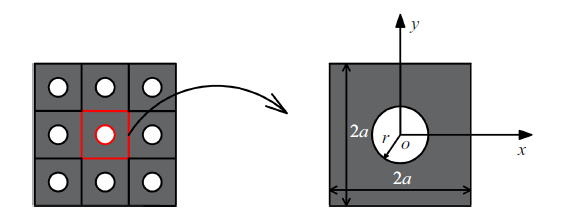Fig.3   Analysis model of periodic plate with opening

### 2.2 准确性分析

2.2.1 人工弹簧刚度取值收敛性分析

### 图4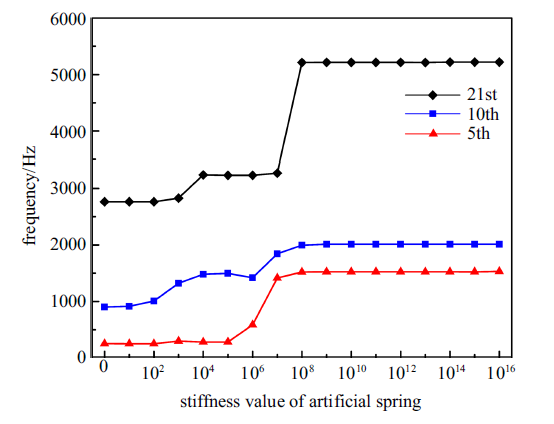Fig.4   Convergence analysis of stiffness of artificial spring

2.2.2 频散特性对比分析

### 图5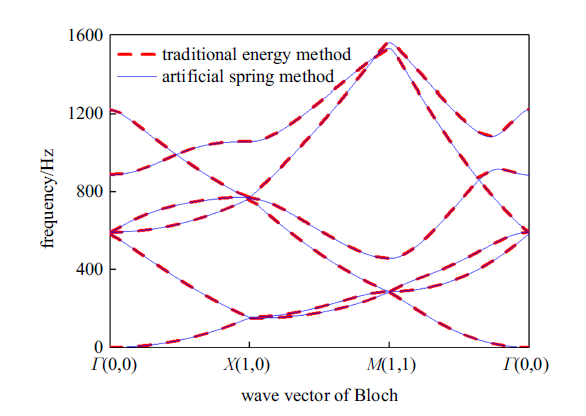Fig.5   Dispersion curve of bending vibration of periodic plate with opening

### 2.3 效率对比分析

2.3.1 扫描波数的影响

Table 1  Comparison of calculation time under different scanning wave number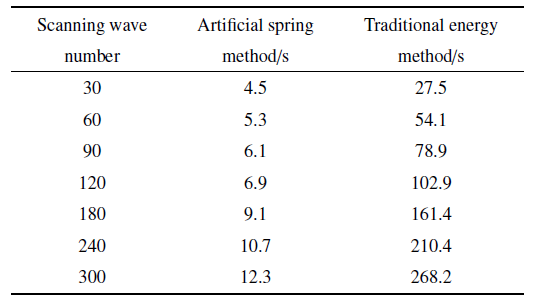2.3.2 矩阵维度的影响

Table 2  Comparison of computational effectiveness under different matrix dimensions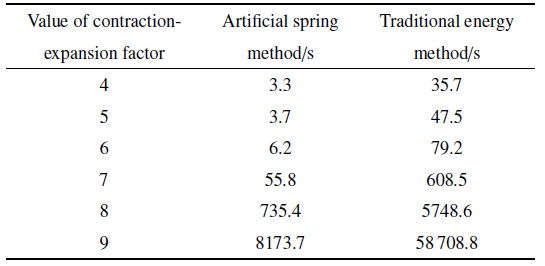## 3 周期组合结构振动带隙计算

### 图6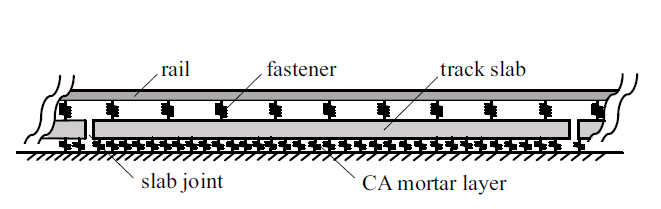Fig.6   Schematic diagram of the longitudinal section of the CRTSIII track structure

### 图7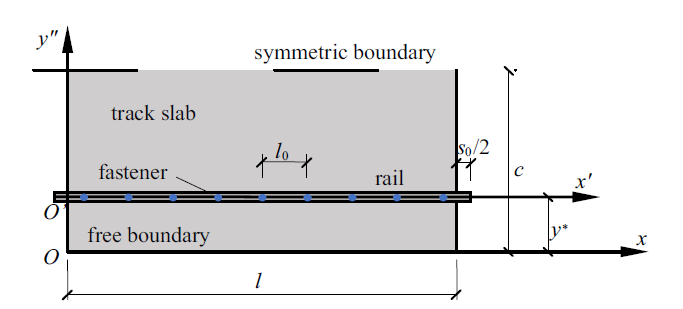Fig.7   Schematic diagram of CRTSIII track structure of a cell

### 3.2 运动方程的建立

$\left. {\begin{array}{l} w_b \left( {{x}',t} \right)= \sum_i {a_{i} \left( t \right)\alpha _{i} \left( {{x}'} \right)} = a^T\alpha =\alpha^T a \\ \theta_b \left( {{x}',t} \right)= \sum_i {b_{i} \left( t \right)\alpha_{i} \left( {{x}'} \right)} = b^T\alpha =\alpha ^T b \\ \end{array}} \right\}$
$\left. {\begin{array}{l} w_{p} \left( {x,y,t} \right)= \sum_i {c_{i} \left( t \right) \beta _{i} \left( {x,y} \right)} = c^T \beta = \beta^T c \\ \theta_{px} \left( {x,y,t} \right)= \sum_i {d_{i} \left( t \right) \beta_{i} \left( {x,y} \right)} = d^T \beta = \beta ^T d \\ \theta_{py} \left( {x,y,t} \right)= \sum_i {e_{i} \left( t \right) \beta_{i} \left( {x,y} \right)} = e^T \beta = \beta ^T e \\ \end{array}} \right\}$

3.2.1 钢轨的动能和应变能

\begin{align} E_{k}^rail=\frac{1}{2}\int_0^{l+s_{0} } {\rho _b A_b \dot{{w}}_b ^{2}d{x}'} +\frac{1}{2}\int_0^{l+s_{0} } {\rho _b I_b \dot{{\theta }}_b^{2}d{x}'} = \\ \frac{1}{2}\dot{{a}}^{H}\left[ {\int_0^{l+s_{0} } {\rho _b A_b \alpha \alpha^{H}d{x}'} } \right]\dot{{a}}+ \\ \frac{1}{2}\dot{{b}}^{H}\left[ {\int_0^{l+s_{0} } {\rho _b I_b \alpha \alpha ^{H}d{x}'} } \right]\dot{{b}} =\frac{1}{2}\dot{{\gamma }}_{1}^{H}M_rail \dot{{\gamma }}_{1} \end{align}
\begin{align} U_{p}^rail=\frac{1}{2}\int_0^{l+s_{0} } {E_b I_b \left( {\frac{\partial \theta_b }{\partial {x}'}} \right)^{2}d{x}'} + \\ \frac{1}{2}\int_0^{l+s_{0} } {\kappa_b G_b A_b \left( {\frac{\partial w_b }{\partial {x}'}-\theta_b } \right)^{2}d{x}'} = \frac{1}{2}\gamma_{1}^{H}K_rail \gamma_{1} \end{align}

3.2.2 轨道板的动能和应变能

\begin{align} E_{k}^plate=\frac{1}{2}\int_0^l \int_0^c \rho _{p} h_{p} \dot{{w}}_{p}^{2}dxdy+ \\ \frac{1}{2}\int_0^l {\int_0^c {\frac{\rho _{p} h_{p}^{3} }{12}\left( {\dot{{\theta }}_{px}^{2} +\dot{{\theta }}_{py}^{2} } \right)dxdy} } = \\ \frac{1}{2}\dot{{c}}^{H}\left[ {\int_0^l {\int_0^c {\rho _{p} h_{p} \beta \beta^{H}dxdy} } } \right]\dot{{c}}+ \\ \frac{1}{2}\dot{{d}}^{H}\left[ {\int_0^l {\int_0^c {\frac{\rho _{p} h_{p}^{3} }{12} \beta \beta ^{H}dxdy} } } \right]\dot{{d}} + \\ \frac{1}{2}\dot{{e}}^{H}\left[ {\int_0^l {\int_0^c {\frac{\rho _{p} h_{p}^{3} }{12} \beta \beta^{H}dxdy} } } \right]\dot{{e}} = \\ \frac{1}{2}\dot{{\gamma }}_{2}^{H}M_plate \dot{{\gamma }}_{2} \end{align}
\begin{align} U_{p}^plate=\frac{1}{2}\int_0^l \int_0^c D_{p} \Bigg[ \left( \frac{\partial \theta_{px} }{\partial x}+\frac{\partial \theta _{py} }{\partial y} \right)^{2}- 2\left( {1-\nu } \right)\cdot \\ \frac{\partial \theta_{px} }{\partial x}\frac{\partial \theta_{py} }{\partial y} +\frac{1}{2}\left( {1-\nu } \right)\left( {\frac{\partial \theta_{px} }{\partial y}+\frac{\partial \theta_{py} }{\partial x}} \right)^{2} \Bigg]dxdy + \\ \frac{1}{2}\int_0^l \int_0^c \kappa_{p} G_{p} A_{p} \Bigg[ \left( {\theta_{px} +\frac{\partial w_{p} }{\partial x}} \right)^{2}+ \\ \left( {\theta_{py} +\frac{\partial w_{p} }{\partial y}} \right)^{2} \Bigg]dxdy =\frac{1}{2}\gamma_{2}^{H}K_plate \gamma_{2} \end{align}

3.2.3 扣件弹性势能与轨道板下弹性地基势能

\begin{align} U_{p}^fastener=\frac{1}{2}\sum_{n=1}^9 k_f \Bigg\{ w_b \left[ {\frac{l_{0} }{2}+\left( {n-1} \right)l_{0} } \right]- \\ w_{p} \left[ \frac{l_{0} -s_{0} }{2}+\left( {n-1} \right)l_{0},\ y^{{\ast }}\right] \Bigg\}^{2} = \\ \frac{1}{2}\gamma^{H}K_fastener \gamma \end{align}

$U_{p}^ca=\frac{1}{2}\int_0^l {\int_0^c {k_ca w_{p}^{2} dxdy} } =\frac{1}{2}\gamma_{2}^{H}K_ca \gamma_{2}$

3.2.4 对称边界及周期性边界条件的定义

$U_{p}^symmetric=\frac{1}{2}\int_0^l[ {k_{ts} } w_{p}^{2} \left( {x,c} \right)+k_{rs} \theta_{py}^{2} \left( {x,c} \right) ]dx= \\ \frac{1}{2}\gamma_{2}^{H}K_symmetric \gamma_{2}$
\begin{align} U_{p}^periodic=\frac{1}{2}\Big\{ k_{tx} \left[ {w_b \left( 0 \right)-w\left( {l+s_{0} } \right)e^{-ik\left( {l+s_{0} } \right)}} \right]^{2} + \\ k_{rx} \left[ {\theta_b \left( 0 \right)-\theta_b \left( {l+s_{0}} \right)e^{-ik\left( {l+s_{0} } \right)}} \right]^{2}\Big\} = \\ \frac{1}{2}\gamma_{1}^{H}K_periodic \left( k \right)\gamma_{1} \end{align}

3.2.5 单个周期的拉格朗日能量泛函

\begin{align} E_{k} =E_{k}^rail+E_{k}^plate=\frac{1}{2}\dot{{\gamma }}_{1} ^{H}M_rail \dot{{\gamma }}_{1} +\frac{1}{2}\dot{{\gamma }}_{2} ^{H}M_plate \dot{{\gamma }}_{2} = \\ \frac{1}{2}\dot{{\gamma }}^{H}\left[ {{\begin{array}{cc} {M_rail } & \\[-2mm] & {M_plate } \\ \end{array} }} \right]\dot{{\gamma }}=\frac{1}{2}\dot{{\gamma }}^{H}M\dot{{\gamma }} \end{align}
\begin{align} U_{p} =U_{p}^rail+U_{p}^plate+U_{p}^fastener+ U_{p} ^{ca}+U_{p}^symmetric+U_{p}^periodic =\\ \frac{1}{2}\gamma^{H}\Bigg\{\Bigg[ \begin{array}{cc} K_rail +K_periodic( k) & \\[-2mm] & {K_plate +K_ca +K_symmetric } \\ \end{array} \Bigg]+ K_fastener \Bigg\}\gamma \end{align}

\begin{align} L=E_{k} -U_{p} =\frac{1}{2}\dot{{\gamma }}^{H}M\dot{{\gamma }}- \\ \frac{1}{2}\gamma^{H}\Bigg\{\Bigg[ \begin{array}{cc} K_rail +K_periodic( k) & \\[-2mm] & {K_plate +K_ca +K_symmetric } \\ \end{array} \Bigg]+ \\ K_fastener \Bigg\}\gamma \end{align}

$\Bigg| \Bigg\{ \Bigg[\begin{array}{*{20}c} {K_rail +K_periodic \left( k \right)} & \\[-2mm] & {K_plate +K_ca +K_symmetric } \\ \end{array} \Bigg]+ \\ K_fastener \Bigg\}-\omega^{2}M \Bigg|=0$

### 图8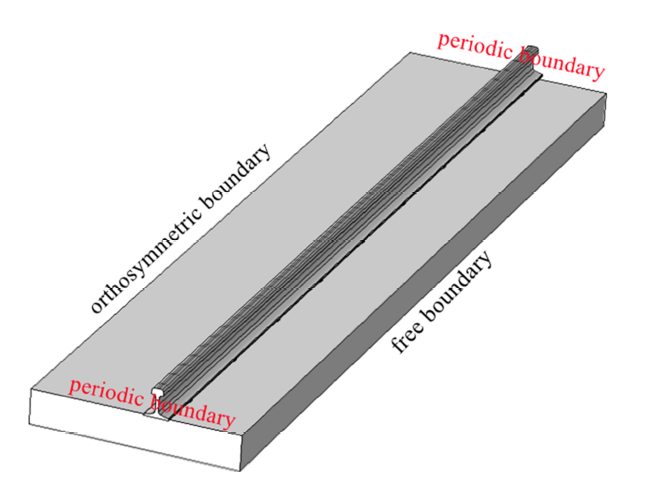Fig.8   FEM model of typical cell of CRTSIII track structure

### 图9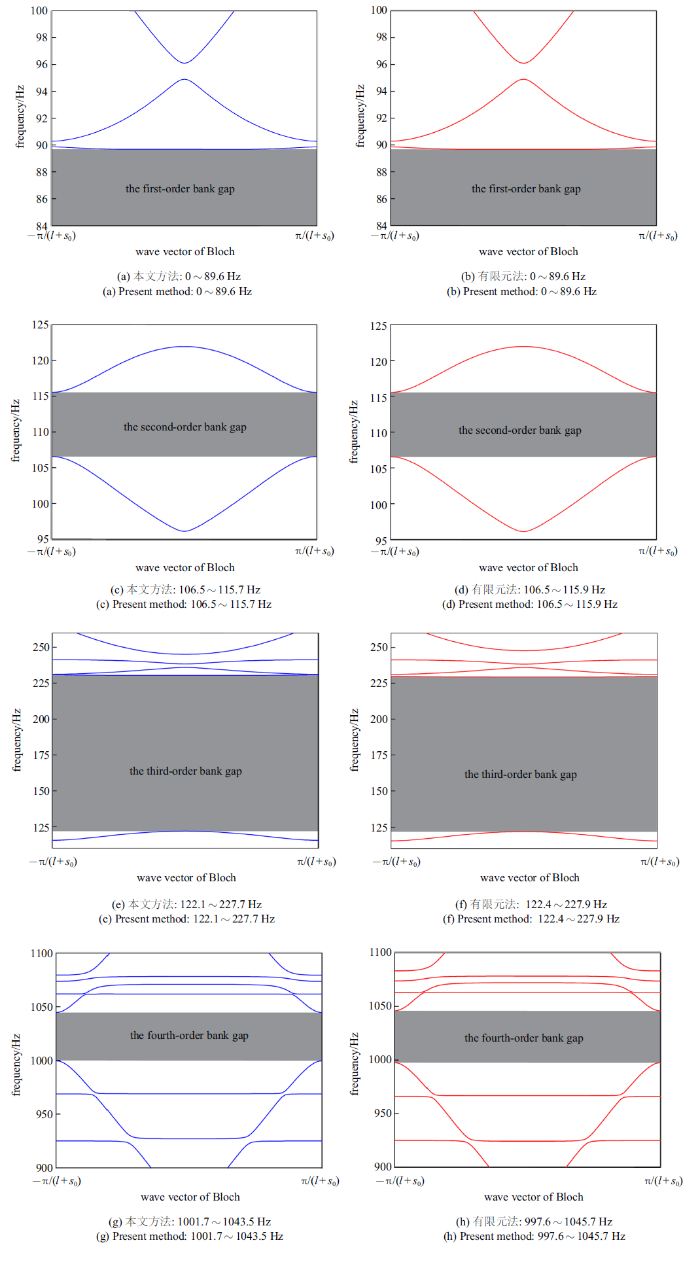Fig.9   Vertical vibration dispersion curve of CRTSIII track structure

## 4 结论

(1) 与传统能量法以及有限元法的对比结果表明了本文方法具有很好的准确性. 同时,相较于传统能量法, 利用人工弹簧模拟周期性边界条件,无需构造满足周期边界的位移形函数, 进一步实现了周期边界与位移形函数的分离,成功解决了传统能量法中构造满足周期性边界条件形函数的困难问题,提高了能量法的适用性.

(2) 与传统能量法相比, 利用人工弹簧模拟周期边界,除周期边界处弹性势能矩阵需随波数变化而重复计算, 其他矩阵均只需计算一次,这极大地提高了计算效率,实现了以较低的计算成本对复杂周期结构的带隙进行精确计算.随着模型复杂程度的增加以及分析精度要求的提高,结构质量、刚度矩阵的维度以及扫描波数点数也随之增多,本文方法在计算效率上的优势会更加明显.

## 参考文献 原文顺序 文献年度倒序 文中引用次数倒序 被引期刊影响因子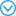(Lu Yiming, Cao Dongxing, Shen Yongjun, et al.

Study on the bandgaps of defect states and application of energy harvesting of local resonant phononic crystal plate

Chinese Journal of Theoretical and Applied Mechanics, 2021, 53(4): 1114-1123 (in Chinese))

Mei J, Wu Y, Liu Z Y.

Effective medium of periodic fluid-solid composites

Europhysics Letters, 2012, 98(5): 54001

(Wen Jihong, Yu Dianlong, Wang Gang, et al.

Elastic wave band gaps in flexural vibration of straight beams

Journal of Mechanical Engineering, 2005, 41(4): 1-6 (in Chinese))

Jr EJPM, Nobrega ED, Rodrigues SF, et al.

Wave attenuation in elastic metamaterial thick plates: Analytical, numerical and experimental investigations

International Journal of Solids and Structures, 2020, 205: 138-152

Wen J, Wang G, Yu D, et al.

Theoretical and experimental investigation of flexural wave propagation in straight beams with periodic structures: Application to a vibration isolation structure

Journal of Applied Physics, 2005, 97: 114907(Chen Ali, Wang Xinmeng, Wang Yuesheng.

Tunable control and functional switch of transmitted acoustic waves by an arch-shaped metasurface

Chinese Journal of Theoretical and Applied Mechanics, 2021, 53(3): 789-801 (in Chinese))

Vinícius Fonseca Dal Poggetto, Serpa AL.

Elastic wave band gaps in a three-dimensional periodic metamaterial using the plane wave expansion method

International Journal of Mechanical Sciences, 2020, 184: 105841

(Chen Shengbing, Han Xiaoyun, Yu Dianlong, et al.

Influences of different types of piezoelectric shunting circuits on band gaps of phononic beam

Acta Physica Sinica, 2010, 59(1): 387-392 (in Chinese))

Qian DH, Wu JH, He FY.

Electro-mechanical coupling band gaps of a piezoelectric phononic crystal Timoshenko nanobeam with surface effects

Ultrasonics, 2021, 109: 106225

Qian DH, Shi ZY, Ning CW, et al.

Nonlinear bandgap properties in a nonlocal piezoelectric phononic crystal nanobeam

Physics Letters A, 2019, 383: 3101-3107

(Xu Zhenlong, Wu Fugen, Huang Liangguo.

Study on low-frequency complete band gaps of local resonant magnetorheological vibration isolators

Piezoelectrics and Acoustooptics, 2015, 37(2): 330-333 (in Chinese))

(Wu Xudong, Zuo Shuguang, Ni Tianxin, et al.

Study on the bandgap characteristics of a locally resonant phononic crystal beam with attached double oscillators in parallel

Journal of Vibration Engineering, 2017, 30(1): 79-85 (in Chinese))

Fomenko SI, Golub MV, Chen A, et al.

Band-gap and pass-band classification for oblique waves propagating in a three-dimensional layered functionally graded piezoelectric phononic crystal

Journal of Sound and Vibration, 2019, 439: 219-240Three-dimensional time-harmonic wave motion in a layered functionally graded (FG) piezoelectric periodic composite (phononic crystal) composed of a finite or an infinite number of unit-cells is considered. A longitudinal or transverse plane waves incident obliquely to the interfaces of a finite phononic crystal between two half-spaces is studied. The paper proposes a semi-analytical method to simulate and analyse the wave fields in a phononic crystal in the case of arbitrary angles of incidence. It is shown that the method is numerically stable for an arbitrary number of unit-cells in finite phononic crystals. Several kinds of pass-bands and band-gaps can be distinguished by employing the derived semi-analytical expressions: band-gaps, pass-bands, low transmission pass-bands, quasi-longitudinal and quasi-transverse band-gaps. Using the present approach a detailed parametric analysis of the influences of the type and incidence angle of an incident wave, and the material and geometrical parameters of the FG interlayers on wave propagation is conducted. (C) 2018 Elsevier Ltd.

Wang T, Sheng MP, et al.

Flexural wave suppression by an acoustic metamaterial plate

Applied Acoustics, 2016, 114: 118-124

Wang T.

Tunable band gaps in an inertant metamaterial plate with two-degree-of-freedom local resonance

Physics Letters A, 2020, 384(21): 126420

Huang ZG, Chen ZY.

Acoustic waves in two-dimensional phononic crystals with reticular geometric structures

Journal of Vibration and Acoustics, 2013, 133(3): 031011

(Zhu Xiaohui, Li Longqiu, Zhang Guangyu, et al.

Investigation of acoustic manipulation by layered periodic composites

Journal of Mechanical Engineering, 2017, 53(6): 10-15 (in Chinese))

Wu LY, Chen LW.

Acoustic band gaps of the woodpile sonic crystal with the simple cubic lattice

Journal of Physics D: Applied Physics, 2011, 44(4): 045402

(Li Jingru, Li Sheng.

Multi-flexural wave band gaps of a new periodic metamaterial plate

Journal of Vibration and Shock, 2018, 37(1): 163-171 (in Chinese))

Hoang T, Duhamel D, Foret G.

Wave finite element method for waveguides and periodic structures subjected to arbitrary loads

Finite Elements in Analysis and Design, 2020, 179: 103437

Jean-Mathieu M.

A wave finite element approach for the analysis of periodic structures with cyclic symmetry in dynamic substructuring

Journal of Sound and Vibration, 2018, 431: 1-17

Nobrega ED, Gautier F, Pelat A, et al.

Vibration band gaps for elastic metamaterial rods using wave finite element method

Mechanical Systems and Signal Processing, 2016, 79(15): 192-202

Zhou CW, Lainé JP, Ichchou MN, et al.

Numerical and experimental investigation on broadband wave propagation features in perforated plates

Mechanical Systems and Signal Processing, 2016, 75: 556-575

Hong J, He X, Zhang D, et al.

Vibration isolation design for periodically stiffened shells by the wave finite element method

Journal of Sound and Vibration, 2018, 419: 90-102

Li FM, Zhang CX, Liu C.

Active tuning of vibration and wave propagation in elastic beams with periodically placed piezoelectric actuator/sensor pairs

Journal of Sound and Vibration, 2017, 393: 14-29

Atari AK, Stephen NG.

On wave propagation in repetitive structures: Two forms of transfer matrix

Journal of Sound and Vibration, 2019, 439: 99-112

(Wu Jian, Bai Xiaochun, Xiao Yong, et al.

Low frequency band gaps and vibration reduction properties of a multi-frequency locally resonant phononic plate

Acta Physica Sinica, 2016, 65(6): 064602 (in Chinese))

Miranda Jr. EJP, Nobrega ED, Ferreira AHR, et al.

Flexural wave band gaps in a multi-resonator elastic metamaterial plate using Kirchhoff-Love theory

Mechanical Systems and Signal Processing, 2019, 116(1): 480-504

Wang P, Yi Q, Zhao C, et al.

Wave propagation in periodic track structures: Band-gap behaviours and formation mechanisms

Archive of Applied Mechanics, 2017, 87(3): 503-519

Wang P, Yi Q, Zhao C, et al.

Elastic wave propagation characteristics of periodic track structure in high-speed railway

Journal of Vibration and Control, 2019, 25(3): 517-528

Jin G, Zhang C, Ye T, et al.

Band gap property analysis of periodic plate structures under general boundary conditions using spectral-dynamic stiffness method

Applied Acoustics, 2017, 121: 1-13

(Yan Zhizhong, Wang Yuesheng.

Wavelet-based method for computing elastic band gaps of one-dimensional phononic crystals

Sci Sin-Phys Mech Astron, 2007, 37(4): 544-551 (in Chinese))

Carter BG, McIver P.

Water-wave propagation through an infinite array of floating structures

Journal of Engineering Mathematics, 2013, 81(1): 9-45

Chen M, Jin G, Zhang Y, et al.

Three-dimensional vibration analysis of beams with axial functionally graded materials and variable thickness

Composite Structures, 2019, 207: 304-322(Hua Hongliang, Liao Zhenqiang, Zhang Xiangyan.

An efficient dynamic modeling method of an axially moving cantilever beam and frequency response analysis

Chinese Journal of Theoretical and Applied Mechanics, 2017, 49(6): 1390-1398 (in Chinese))

Qu Y, Wu S, Chen Y, et al.

Vibration analysis of ring-stiffened conical-cylindrical-spherical shells based on a modified variational approach

International Journal of Mechanical Sciences, 2013, 69: 72-84

Qu Y, Chen Y, Long X, et al.

A modified variational approach for vibration analysis of ring-stiffened conical-cylindrical shell combinations

European Journal of Mechanics, A/Solids, 2013, 37: 200-215

Wang T, Sheng MP, Qin QH.

Multi-flexural band gaps in an Euler-Bernoulli beam with lateral local resonators

Physics Letters A, 2016, 380(4): 525-529

Wang T, Qin QH, Zhu X.

Reaction force and power flow analysis of an acoustic metamaterial beam with multi-band gaps

Acoustics Australia, 2020, 48(1): 59-67

Tang L, Cheng L.

Broadband locally resonant band gaps in periodic beam structures with embedded acoustic black holes

Journal of Applied Physics, 2017, 121: 194901

Deng J, Guasch O, Zheng L.

A semi-analytical method for characterizing vibrations in circular beams with embedded acoustic black holes

Journal of Sound and Vibration, 2020, 476: 115307

Jin G, Ye T, Wang X, et al.

A unified solution for the vibration analysis of FGM doubly-curved shells of revolution with arbitrary boundary conditions

Compos Part B-Eng, 2016, 89: 230-252

Zhang C, Jin G, Ma X, et al.

Vibration analysis of circular cylindrical double-shell structures under general coupling and end boundary conditions

Applied Acoustics, 2016, 110: 176-193

Chen Y, Ye T, Jin G.

Quasi-3D solutions for the vibration of solid and hollow slender structures with general boundary conditions

Computers & Structures, 2018, 211: 14-26

Deng J, Zheng L, Guasch O, et al.

Gaussian expansion for the vibration analysis of plates with multiple acoustic black holes indentations

Mechanical Systems and Signal Processing, 2019, 131: 317-334The acoustic black hole (ABH) effect can be achieved embedding cuneate indentations with power-law profile in plates. That results in remarkable properties like the reduction of plate vibrations, the potential for energy harvesting thanks to the focalization of energy, or the design of new metamaterials to manipulate acoustics waves. The analysis of such phenomena demands simulating the modal shapes and response to external excitations of ABH plates. This is usually done by means of numerical approaches, like the finite element method (FEM). However, if one is interested in performing long parametric analyses and in capturing the ABH plate behavior at high frequencies, the computational cost associated to numerical methods may become too demanding. In this work, a semi-analytical approach is suggested to circumvent the situation. The Rayleigh-Ritz method is applied using two-dimensional Gaussian functions to expand the flexural motion of a plate with non-uniform thickness. Then, a matrix-replacing strategy is proposed to embed the multiple ABHs in the plate. That results in low dimensional matrix systems, which yet provide very accurate solutions. After presenting all theoretical developments, the semi-analytical method is first applied to analyze the performance of a single ABH when varying several of its parameters. Then, various configurations involving multiple ABHs are considered. Those range from long strips exhibiting frequency attenuation bands, to plates containing ABH indentations in many shapes and sizes. (C) 2019 Elsevier Ltd. All rights reserved.

Deng J, Zheng L, Zeng P, et al.

Passive constrained viscoelastic layers to improve the efficiency of truncated acoustic black holes in beams

Mechanical Systems and Signal Processing, 2018, 118: 461-476

/

 〈〉